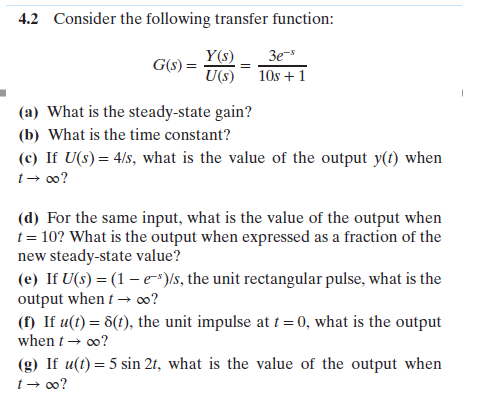# 4.2Consider the following transfer function:Y(s) 3e-U(s) 1Os +1(a) What is the steady-state gain?(b) What is the time constant?(c) If U(s)4/s, what is the value of the output y(t) whent oo?(d) For the same input, what is the value of the output whent 10? What is the output when expressed as a fraction of thenew steady-state value?(e) If U(s) (1-e-)s, the unit rectangular pulse, what is theoutput whent-oo?( If u() 6(), the unit impulse at 0, what is the outputwhen-00?(g) If u(t) 5 sin 2t, what is the value of the output when

Questionhelp_outlineImage Transcriptionclose4.2 Consider the following transfer function: Y(s) 3e- U(s) 1Os +1 (a) What is the steady-state gain? (b) What is the time constant? (c) If U(s)4/s, what is the value of the output y(t) when t oo? (d) For the same input, what is the value of the output when t 10? What is the output when expressed as a fraction of the new steady-state value? (e) If U(s) (1-e-)s, the unit rectangular pulse, what is the output whent-oo? ( If u() 6(), the unit impulse at 0, what is the output when-00? (g) If u(t) 5 sin 2t, what is the value of the output when fullscreen
check_circle

Step 1

Part g:

The part can be solved in two ways:

• Apply final value theorem and find the ultimate value of the response. First find the Laplace transform of the input function u(t)=5sin2t and then put the final value theorem and the limits s tends to 0 in the function.
• Find the response or output in terms of t by using inverse transform of Y(s) function and then put the value to t to infinite.

We are using method (1) in order to find the value of output at t tends to infinite.

The Laplace transform of the input function is

Step 2

Hence the response is

Step 3

According to the final value the...

### Want to see the full answer?

See Solution

#### Want to see this answer and more?

Solutions are written by subject experts who are available 24/7. Questions are typically answered within 1 hour.*

See Solution
*Response times may vary by subject and question.
Tagged in

### Chemical Engineering